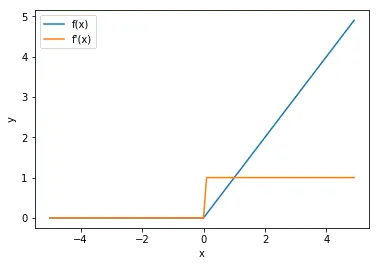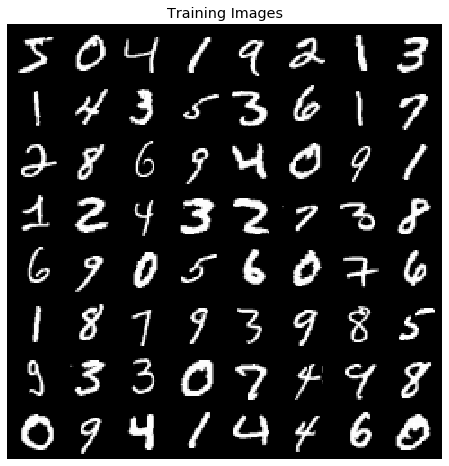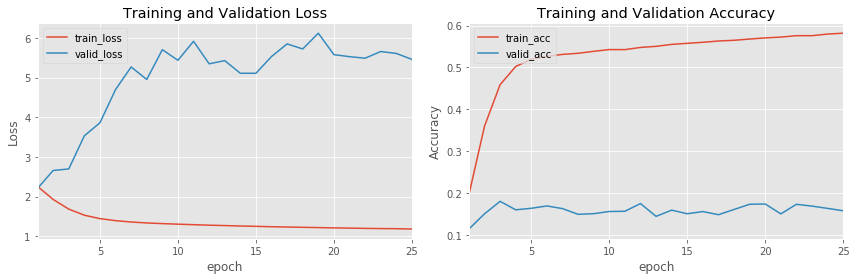# Activation Functions¶

In D.L., our objective is, almost always, to find a set of weights that minimizes error. All of these sets of weights are linear operations and hence, if performed alone, we would attain just a simple multiple linear regression model.

##### What’s the Problem with Linear Models?¶

If inputs are left untouched, they are not flexible as they can only model linear relationships while most data out there has a non-linear patterns. Hence, we need to find a way to force our model to be able to learn non-linear patterns.

##### How do we do this?¶

After a set of linear operations, we apply to the new input created by the linear operations ($Ax = \hat{y}$) a non-linear activation function.

Suppose we have a simple linear model $\hat{y}=ax+b$. These $\hat{y}$ form a linear operation such as below:

Well, given our orange line ($\hat{y}$), we then apply a non-linear activation function so as to transform our linear model into a fixed non-linear model such as below:Why is this a fixed non-linear operation?

Because whatever formula we use for our non-linear operation, we do NOT have a set of weights on it that try to learn an optimal non-linear representation. It will always follow a fixed, single transformation.

##### Well, isn’t our purpose to find an optimal non-linear operation?¶

Yes and no. We find an optimal non-linear operation by letting our set of linear weights learn a representation of the data that, once fed to the non-linear operation, will correctly identify the new pattern. Hence, the objective of our linear weights now becomes to find a representation of the data that, once fed to the non-linear activation, will correctly learn the non-linear patterns.

##### How do we backpropagate these non-linear activations?¶

Given that these non-linear activations are in fact non-linear, we are unable to just take the input as the gradient as we can do with linear operations (3x => 3). Hence, we will need two things:

1. The input ($\hat{y}$) that was fed to the non-linear activation and
2. The derivative equation of the non-linear function.

Given that we want to apply the non-linear operation to every input, we can classify these operations as element-wise.

This has important implications on how we can calculate our gradient.

First, as we learned on the "Linear Layer" tutorial, the dimension of the incoming gradient from our subsequent operation will equal the dimension of the output from our non-linear operation.

Now, since the output of the non-linear operations equals the dimension of the input, we are able to calculate the corresponding chain-gradient with a simple Hadamard product (element-wise multiplication) between our incoming gradient and our current non-linear operation. In other words,

input.shape == output.shape == incoming_grad.shape

Second, given that there are no weight parameters to these operations holds two implications:

i) from a backward perspective, these operations are only intermediate variables and

ii) we can just apply the derivative of the equation to each input such as shown below

$$z = \sigma(y)=\sigma(x_ow_0+x_1w_1+x_2w_2+x_3w_3) = \sigma(x_ow_0)+\sigma(x_1w_1)+\sigma(x_2w_2)+\sigma(x_3w_3)$$

Hence

$$\frac{\partial z}{\partial y} = \frac{\partial z}{\partial y}(x_ow_0+x_1w_1+x_2w_2+x_3w_3) = \frac{\partial z}{\partial y}(x_ow_0)+\frac{\partial z}{\partial y}(x_1w_1)+\frac{\partial z}{\partial y}(x_2w_2)+\frac{\partial z}{\partial y}(x_3w_3)$$

Now that we generally understand how to implement non-linear operations, it begs to ask, what are some common non-linear operations?

Some common activation functions are shown below:

• ReLU
• Hyperbolic Tangent (tanh)
• Leaky ReLU

Each has their own unique properties and usually, finding the best best corresponding non-linear activation to a model is left to trial and error.

# ReLU¶

In this tutorial, we will first focus on implementing the ReLU layer and towards the end, for comparison purposes, we will define alternative activation functions.

ReLU is a piece-wise linear, vector valued function that adds non-linearity to our model. The effects that this simple piece-wise function has had on the DL sphere have been astonishing.

The ReLU's forward and backward pass can both be seen as "gates" that either inhibit or advance the flow of either operations.

During the forward pass, ReLU either retains the original content of the input if its greater than zero or else, turns it to zero.

[x if x > 0 else 0 for x in input]


For the inputs that were "cut" to zero, its gradients are turned to zero while the rest of the values become 1. Hence, and given that ReLU is an intermediate operation, ReLU either restricts values of the incoming gradients or lets them "flow". This process is graphed below:Such simple conditions make ReLU a "lightweight" operation as it does not take much to compute its forward and backward method

Such properties, and its surprising effectiveness to model non-linearity, have made ReLU a very popular choice of option for most DL architectures.

Let us model this process in PyTorch

In :
import torch
import torch.nn as nn
torch.randn((2,2)).cuda()

Out:
tensor([[ 1.6169, -0.8602],
[ 0.2214, -0.4084]], device='cuda:0')
In :
# custom ReLU function
# Remember that:
# input.shape == out.shape == incoming_gradient.shape

@staticmethod
def forward(self, input):
# save input for backward() pass
self.save_for_backward(input) # wraps in a tuple structure
activated_input = torch.clamp(input, min = 0)
return activated_input

@staticmethod
"""
In the backward pass we receive a Tensor containing the
gradient of the loss with respect to our f(x) output,
and we now need to compute the gradient of the loss
wrt the input.
"""
# keep in mind that the gradient of ReLU is binary = {0,1}
# hence, we will either keep the element of the output_grad_wrt_loss
# or turn it to zero
input, = self.saved_tensors

In :
# Wrap ReLU_layer function in nn.module
class ReLU(nn.Module):
def __init__(self):
super().__init__()

def forward(self, input):
output = ReLU_layer.apply(input)
return output


In :
# test function with linear + relu layer
dummy_input= torch.ones((1,2)) # input

# forward pass
linear = nn.Linear(2,3)
relu = ReLU()
linear2 = nn.Linear(3,1)

output1 = linear(dummy_input)
output2 = relu(output1)
output3 = linear2(output2)
output3

Out:
tensor([[0.2316]], grad_fn=<AddmmBackward>)
In :
# backward pass
output3.backward()

In :
# check computed gradients of 1st linear layaer

Out:
tensor([[0.1558, 0.1558],
[0.0000, 0.0000],
[0.0000, 0.0000]])

# MNIST¶

Now that we have validated our operation, let's us apply ReLU to the MNIST dataset by building a standard neural network with the following linear parameters:

[128, 64, 10]

In :
class NeuralNet(nn.Module):
def __init__(self, num_units = 128, activation = ReLU()):
super().__init__()

# fully-connected layers
self.fc1 = nn.Linear(784,num_units)
self.fc2 = nn.Linear(num_units , num_units//2)
self.fc3 = nn.Linear(num_units // 2, 10)

# init activation
self.activation = activation

def forward(self,x):

# 1st layer
output = self.activation(self.fc1(x))

# 2nd layer
output = self.activation(self.fc2(output))

# 3rd layer
output = self.fc3(output)

# output.shape = (B, 10)
return output


In :
# instantiate model and feed it to GPU
device = torch.device('cuda')
model = NeuralNet().to(device)
model

Out:
NeuralNet(
(fc1): Linear(in_features=784, out_features=128, bias=True)
(fc2): Linear(in_features=128, out_features=64, bias=True)
(fc3): Linear(in_features=64, out_features=10, bias=True)
(activation): ReLU()
)
In :
# define optimizer
from torch import optim
optimizer = optim.SGD(model.parameters(), lr = .01)

In :
# define criterion
criterion = nn.CrossEntropyLoss()

In :
# import training MNIST dataset
import torchvision
from torchvision import transforms
import numpy as np
from torchvision.utils import make_grid
import matplotlib.pyplot as plt
plt.style.use('ggplot')

root = r'C:\Users\erick\PycharmProjects\untitled\3D_2D_GAN\MNIST_experimentation'
train_mnist = torchvision.datasets.MNIST(root = root,
train = True,
transform = transforms.ToTensor(),
)

train_mnist.data.shape

Out:
torch.Size([60000, 28, 28])
In :
# import evaluation MNIST dataset

eval_mnist = torchvision.datasets.MNIST(root = root,
train = False,
transform = transforms.ToTensor(),
)
eval_mnist.data.shape

Out:
torch.Size([10000, 28, 28])
In :
# visualize data
# visualize our data

grid_images = np.transpose(make_grid(train_mnist.data[:64].unsqueeze(1)), (1,2,0))
plt.figure(figsize=(8,8))
plt.axis("off")
plt.title("Training Images")
plt.imshow(grid_images,cmap = 'gray')

Out:
<matplotlib.image.AxesImage at 0x1cb924fc2e8>In :
# normalize data
train_mnist.data = (train_mnist.data.float() - train_mnist.data.float().mean()) / train_mnist.data.float().std()
eval_mnist.data = (eval_mnist.data.float() - eval_mnist.data.float().mean()) / eval_mnist.data.float().std()

In :
# parse data to batches of 128

# pin_memory = True if you have CUDA. It will speed up I/O

train_dl = DataLoader(train_mnist, batch_size = 64,
shuffle = True, pin_memory = True)

eval_dl = DataLoader(eval_mnist, batch_size = 128,
shuffle = True, pin_memory = True)

batch_images, batch_labels = next(iter(train_dl))
print(f"batch_images.shape: {batch_images.shape}")
print('-'*50)
print(f"batch_labels.shape: {batch_labels.shape}")

batch_images.shape: torch.Size([64, 1, 28, 28])
--------------------------------------------------
batch_labels.shape: torch.Size()


# Train Neural Net¶

In :
# compute average accuracy of batch

def accuracy(pred, labels):
# predictions.shape = (B, 10)
# labels.shape = (B)

n_batch = labels.shape

# extract idx of max value from our batch predictions
# predicted.shape = (B)
_, preds = torch.max(pred, 1)

# compute average accuracy of our batch
compare = (preds == labels).sum()
return compare.item() / n_batch


In :
def train(model, iterator, optimizer, criterion):

# hold avg loss and acc sum of all batches
epoch_loss = 0
epoch_acc = 0

for batch in iterator:

# zero-out all gradients (if any) from our model parameters

# extract input and label

# input.shape = (B, 784), "flatten" image
input = batch.view(-1,784).cuda().float() # shape: (B, 784), "flatten" image
# label.shape = (B)
label = batch.cuda()

# Start PyTorch's Dynamic Graph

# predictions.shape = (B, 10)
predictions = model(input)

# average batch loss
loss = criterion(predictions, label)

# "clears" PyTorch's dynamic graph
loss.backward()

# perform SGD "step" operation
optimizer.step()

# Given that PyTorch variables are "contagious" (they record all operations)
# we need to ".detach()" to stop them from recording any performance
# statistics

# average batch accuracy
acc = accuracy(predictions.detach(), label)

# record our stats
epoch_loss += loss.detach()
epoch_acc += acc

# NOTE: tense.item() unpacks Tensor item to a regular python object
# tense.tensor().item() == 1

# return average loss and acc of epoch
return epoch_loss.item() / len(iterator), epoch_acc / len(iterator)

In :
def evaluate(model, iterator, criterion):

epoch_loss = 0
epoch_acc = 0

# turn off grad tracking as we are only evaluation performance

for batch in iterator:

# extract input and label
input = batch.view(-1,784).cuda()
label = batch.cuda()

# predictions.shape = (B, 10)
predictions = model(input)

# average batch loss
loss = criterion(predictions, label)

# average batch accuracy
acc = accuracy(predictions, label)

epoch_loss += loss
epoch_acc += acc

return epoch_loss.item() / len(iterator), epoch_acc / len(iterator)

In :
import time

# record time it takes to train and evaluate an epoch
def epoch_time(start_time, end_time):
elapsed_time = end_time - start_time # total time
elapsed_mins = int(elapsed_time / 60) # minutes
elapsed_secs = int(elapsed_time - (elapsed_mins * 60)) # seconds
return elapsed_mins, elapsed_secs

In :
N_EPOCHS = 25

# track statistics
track_stats = {'activation': [],
'epoch': [],
'train_loss': [],
'train_acc': [],
'valid_loss':[],
'valid_acc':[]}

best_valid_loss = float('inf')

for epoch in range(N_EPOCHS):

start_time = time.time()

train_loss, train_acc = train(model, train_dl, optimizer, criterion)
valid_loss, valid_acc = evaluate(model, eval_dl, criterion)

end_time = time.time()

# record operations
track_stats['activation'].append('ReLU')
track_stats['epoch'].append(epoch + 1)
track_stats['train_loss'].append(train_loss)
track_stats['train_acc'].append(train_acc)
track_stats['valid_loss'].append(valid_loss)
track_stats['valid_acc'].append(valid_acc)

epoch_mins, epoch_secs = epoch_time(start_time, end_time)

# if this was our best performance, record model parameters
if valid_loss < best_valid_loss:
best_valid_loss = valid_loss
torch.save(model.state_dict(), 'best_linear_relu_params.pt')

# print out stats
print('-'*75)
print(f'Epoch: {epoch+1:02} | Epoch Time: {epoch_mins}m {epoch_secs}s')
print(f'\tTrain Loss: {train_loss:.3f} | Train Acc: {train_acc*100:.2f}%')
print(f'\t Val. Loss: {valid_loss:.3f} |  Val. Acc: {valid_acc*100:.2f}%')

---------------------------------------------------------------------------
Epoch: 01 | Epoch Time: 0m 12s
Train Loss: 2.257 | Train Acc: 19.67%
Val. Loss: 2.208 |  Val. Acc: 11.47%
---------------------------------------------------------------------------
Epoch: 02 | Epoch Time: 0m 15s
Train Loss: 1.928 | Train Acc: 35.99%
Val. Loss: 2.658 |  Val. Acc: 15.09%
---------------------------------------------------------------------------
Epoch: 03 | Epoch Time: 0m 17s
Train Loss: 1.684 | Train Acc: 45.90%
Val. Loss: 2.697 |  Val. Acc: 18.07%
---------------------------------------------------------------------------
Epoch: 04 | Epoch Time: 0m 17s
Train Loss: 1.530 | Train Acc: 50.23%
Val. Loss: 3.533 |  Val. Acc: 16.05%
---------------------------------------------------------------------------
Epoch: 05 | Epoch Time: 0m 16s
Train Loss: 1.444 | Train Acc: 51.98%
Val. Loss: 3.861 |  Val. Acc: 16.42%
---------------------------------------------------------------------------
Epoch: 06 | Epoch Time: 0m 17s
Train Loss: 1.393 | Train Acc: 52.64%
Val. Loss: 4.699 |  Val. Acc: 16.97%
---------------------------------------------------------------------------
Epoch: 07 | Epoch Time: 0m 17s
Train Loss: 1.359 | Train Acc: 53.11%
Val. Loss: 5.268 |  Val. Acc: 16.34%
---------------------------------------------------------------------------
Epoch: 08 | Epoch Time: 0m 16s
Train Loss: 1.335 | Train Acc: 53.38%
Val. Loss: 4.952 |  Val. Acc: 14.97%
---------------------------------------------------------------------------
Epoch: 09 | Epoch Time: 0m 17s
Train Loss: 1.319 | Train Acc: 53.84%
Val. Loss: 5.703 |  Val. Acc: 15.13%
---------------------------------------------------------------------------
Epoch: 10 | Epoch Time: 0m 17s
Train Loss: 1.304 | Train Acc: 54.26%
Val. Loss: 5.436 |  Val. Acc: 15.64%
---------------------------------------------------------------------------
Epoch: 11 | Epoch Time: 0m 17s
Train Loss: 1.292 | Train Acc: 54.25%
Val. Loss: 5.915 |  Val. Acc: 15.70%
---------------------------------------------------------------------------
Epoch: 12 | Epoch Time: 0m 17s
Train Loss: 1.279 | Train Acc: 54.77%
Val. Loss: 5.348 |  Val. Acc: 17.54%
---------------------------------------------------------------------------
Epoch: 13 | Epoch Time: 0m 18s
Train Loss: 1.269 | Train Acc: 55.03%
Val. Loss: 5.428 |  Val. Acc: 14.49%
---------------------------------------------------------------------------
Epoch: 14 | Epoch Time: 0m 17s
Train Loss: 1.258 | Train Acc: 55.50%
Val. Loss: 5.109 |  Val. Acc: 15.97%
---------------------------------------------------------------------------
Epoch: 15 | Epoch Time: 0m 18s
Train Loss: 1.250 | Train Acc: 55.75%
Val. Loss: 5.109 |  Val. Acc: 15.11%
---------------------------------------------------------------------------
Epoch: 16 | Epoch Time: 0m 18s
Train Loss: 1.240 | Train Acc: 56.00%
Val. Loss: 5.531 |  Val. Acc: 15.63%
---------------------------------------------------------------------------
Epoch: 17 | Epoch Time: 0m 18s
Train Loss: 1.232 | Train Acc: 56.31%
Val. Loss: 5.849 |  Val. Acc: 14.88%
---------------------------------------------------------------------------
Epoch: 18 | Epoch Time: 0m 18s
Train Loss: 1.225 | Train Acc: 56.48%
Val. Loss: 5.722 |  Val. Acc: 16.13%
---------------------------------------------------------------------------
Epoch: 19 | Epoch Time: 0m 18s
Train Loss: 1.218 | Train Acc: 56.77%
Val. Loss: 6.119 |  Val. Acc: 17.38%
---------------------------------------------------------------------------
Epoch: 20 | Epoch Time: 0m 20s
Train Loss: 1.211 | Train Acc: 57.05%
Val. Loss: 5.578 |  Val. Acc: 17.43%
---------------------------------------------------------------------------
Epoch: 21 | Epoch Time: 0m 18s
Train Loss: 1.205 | Train Acc: 57.24%
Val. Loss: 5.528 |  Val. Acc: 15.08%
---------------------------------------------------------------------------
Epoch: 22 | Epoch Time: 0m 18s
Train Loss: 1.198 | Train Acc: 57.58%
Val. Loss: 5.489 |  Val. Acc: 17.37%
---------------------------------------------------------------------------
Epoch: 23 | Epoch Time: 0m 18s
Train Loss: 1.193 | Train Acc: 57.58%
Val. Loss: 5.657 |  Val. Acc: 16.93%
---------------------------------------------------------------------------
Epoch: 24 | Epoch Time: 0m 18s
Train Loss: 1.191 | Train Acc: 57.96%
Val. Loss: 5.609 |  Val. Acc: 16.39%
---------------------------------------------------------------------------
Epoch: 25 | Epoch Time: 0m 18s
Train Loss: 1.182 | Train Acc: 58.17%
Val. Loss: 5.460 |  Val. Acc: 15.82%


# Visualization¶

From the above, we can tell that our model is severely suffering from overfitting by the gap between training and validation accuracy. However, to attain a better understanding, we will graph our recorded statistics and use HiPlot, a new graphing library by Facebook, to understand the overall patterns of our model

NOTE: If you do not have HiPlot installed, go to their github repo to find latest installation

In :
# save statistics
#torch.save(track_stats, 'ReLU_stats.pt')

In :
# format data
import pandas as pd

stats = pd.DataFrame(track_stats)
stats

Out:
activation epoch train_loss train_acc valid_loss valid_acc
0 ReLU 1 2.256706 0.196678 2.207836 0.114715
1 ReLU 2 1.928205 0.359941 2.658292 0.150910
2 ReLU 3 1.684190 0.459005 2.697213 0.180676
3 ReLU 4 1.530393 0.502265 3.532650 0.160502
4 ReLU 5 1.443833 0.519773 3.861390 0.164161
5 ReLU 6 1.393192 0.526353 4.698839 0.169699
6 ReLU 7 1.358555 0.531100 5.268126 0.163370
7 ReLU 8 1.335125 0.533765 4.952165 0.149723
8 ReLU 9 1.318727 0.538430 5.703450 0.151305
9 ReLU 10 1.303947 0.542644 5.436379 0.156448
10 ReLU 11 1.291508 0.542494 5.915389 0.157041
11 ReLU 12 1.278763 0.547708 5.347536 0.175435
12 ReLU 13 1.269061 0.550323 5.427631 0.144877
13 ReLU 14 1.257962 0.554971 5.108685 0.159711
14 ReLU 15 1.249527 0.557536 5.108813 0.151108
15 ReLU 16 1.239964 0.559968 5.530833 0.156349
16 ReLU 17 1.232409 0.563083 5.849154 0.148833
17 ReLU 18 1.224834 0.564765 5.722316 0.161294
18 ReLU 19 1.218170 0.567697 6.119403 0.173754
19 ReLU 20 1.210809 0.570496 5.578406 0.174347
20 ReLU 21 1.204878 0.572378 5.527742 0.150811
21 ReLU 22 1.198037 0.575760 5.488918 0.173655
22 ReLU 23 1.193254 0.575776 5.657108 0.169304
23 ReLU 24 1.190567 0.579608 5.608899 0.163865
24 ReLU 25 1.182364 0.581690 5.459799 0.158228

Compare training vs validation statistics

In :
fig, axes = plt.subplots(nrows=1, ncols = 2,figsize = (12,4))
stats[['epoch','train_loss','valid_loss']].plot(x = 'epoch',ax=axes)
axes.title.set_text('Training and Validation Loss')
axes.set_ylabel('Loss')
stats[['epoch','train_acc','valid_acc']].plot(x = 'epoch',ax = axes)
axes.title.set_text('Training and Validation Accuracy')
axes.set_ylabel('Accuracy')
plt.tight_layout()
plt.legend(loc = 'upper left')
plt.show()

<Figure size 1296x1296 with 0 Axes>From the above, we can confidently conclude that our model is severely suffering from overfitting due to the large gap in loss and accuracy statistics.

Now, let us use HiPlot to attain a more complete picture of our model's performance

In :
# organize data to hiplot format

data = []
for row in stats.iterrows():
data.append(row.to_dict())
data

Out:
[{'activation': 'ReLU',
'epoch': 1,
'train_loss': 2.2567057985740937,
'train_acc': 0.1966784381663113,
'valid_loss': 2.20783610283574,
'valid_acc': 0.11471518987341772},
{'activation': 'ReLU',
'epoch': 2,
'train_loss': 1.9282052176339286,
'train_acc': 0.3599413646055437,
'valid_loss': 2.658291973645174,
'valid_acc': 0.15090981012658228},
{'activation': 'ReLU',
'epoch': 3,
'train_loss': 1.6841899164195762,
'train_acc': 0.459005197228145,
'valid_loss': 2.697213281559039,
'valid_acc': 0.18067642405063292},
{'activation': 'ReLU',
'epoch': 4,
'train_loss': 1.5303927748950559,
'train_acc': 0.5022654584221748,
'valid_loss': 3.5326503319076346,
'valid_acc': 0.16050237341772153},
{'activation': 'ReLU',
'epoch': 5,
'train_loss': 1.4438330806902986,
'train_acc': 0.5197727878464818,
'valid_loss': 3.8613895464547072,
'valid_acc': 0.16416139240506328},
{'activation': 'ReLU',
'epoch': 6,
'train_loss': 1.3931915998967217,
'train_acc': 0.5263526119402985,
'valid_loss': 4.698839404914953,
'valid_acc': 0.16969936708860758},
{'activation': 'ReLU',
'epoch': 7,
'train_loss': 1.358555124766791,
'train_acc': 0.5311000799573561,
'valid_loss': 5.268125509913964,
'valid_acc': 0.16337025316455697},
{'activation': 'ReLU',
'epoch': 8,
'train_loss': 1.3351253029634196,
'train_acc': 0.5337653251599147,
'valid_loss': 4.9521654346321204,
'valid_acc': 0.14972310126582278},
{'activation': 'ReLU',
'epoch': 9,
'train_loss': 1.3187274078824627,
'train_acc': 0.5384295042643923,
'valid_loss': 5.703450263301028,
'valid_acc': 0.15130537974683544},
{'activation': 'ReLU',
'epoch': 10,
'train_loss': 1.3039471396505198,
'train_acc': 0.5426439232409381,
'valid_loss': 5.4363789618769776,
'valid_acc': 0.15644778481012658},
{'activation': 'ReLU',
'epoch': 11,
'train_loss': 1.2915082008345549,
'train_acc': 0.5424940031982942,
'valid_loss': 5.915389435200751,
'valid_acc': 0.15704113924050633},
{'activation': 'ReLU',
'epoch': 12,
'train_loss': 1.2787634355427107,
'train_acc': 0.5477078891257996,
'valid_loss': 5.347536111179786,
'valid_acc': 0.17543512658227847},
{'activation': 'ReLU',
'epoch': 13,
'train_loss': 1.269061058060701,
'train_acc': 0.5503231609808102,
'valid_loss': 5.427630847013449,
'valid_acc': 0.14487737341772153},
{'activation': 'ReLU',
'epoch': 14,
'train_loss': 1.257962289903718,
'train_acc': 0.5549706823027718,
'valid_loss': 5.108685457253758,
'valid_acc': 0.1597112341772152},
{'activation': 'ReLU',
'epoch': 15,
'train_loss': 1.2495273354211087,
'train_acc': 0.5575359808102346,
'valid_loss': 5.108813322043117,
'valid_acc': 0.15110759493670886},
{'activation': 'ReLU',
'epoch': 16,
'train_loss': 1.2399636860341152,
'train_acc': 0.5599680170575693,
'valid_loss': 5.530833183964597,
'valid_acc': 0.15634889240506328},
{'activation': 'ReLU',
'epoch': 17,
'train_loss': 1.2324086008295576,
'train_acc': 0.5630830223880597,
'valid_loss': 5.8491543154173256,
'valid_acc': 0.14883306962025317},
{'activation': 'ReLU',
'epoch': 18,
'train_loss': 1.224833994786114,
'train_acc': 0.5647654584221748,
'valid_loss': 5.7223159210591374,
'valid_acc': 0.16129351265822786},
{'activation': 'ReLU',
'epoch': 19,
'train_loss': 1.2181698406683101,
'train_acc': 0.5676972281449894,
'valid_loss': 6.119402535354035,
'valid_acc': 0.17375395569620253},
{'activation': 'ReLU',
'epoch': 20,
'train_loss': 1.210808662463353,
'train_acc': 0.5704957356076759,
'valid_loss': 5.5784058389784414,
'valid_acc': 0.17434731012658228},
{'activation': 'ReLU',
'epoch': 21,
'train_loss': 1.2048782316098081,
'train_acc': 0.572378065031983,
'valid_loss': 5.527741637410997,
'valid_acc': 0.150810917721519},
{'activation': 'ReLU',
'epoch': 22,
'train_loss': 1.1980372186916977,
'train_acc': 0.5757595948827292,
'valid_loss': 5.488918256156052,
'valid_acc': 0.17365506329113925},
{'activation': 'ReLU',
'epoch': 23,
'train_loss': 1.193253962470016,
'train_acc': 0.5757762526652452,
'valid_loss': 5.657107968873616,
'valid_acc': 0.16930379746835442},
{'activation': 'ReLU',
'epoch': 24,
'train_loss': 1.190566984066831,
'train_acc': 0.5796075426439232,
'valid_loss': 5.608898694002176,
'valid_acc': 0.16386471518987342},
{'activation': 'ReLU',
'epoch': 25,
'train_loss': 1.1823643275669642,
'train_acc': 0.5816897654584222,
'valid_loss': 5.459799464744858,
'valid_acc': 0.15822784810126583}]
In :
import hiplot as hip
hip.Experiment.from_iterable(data).display(force_full_width = False)